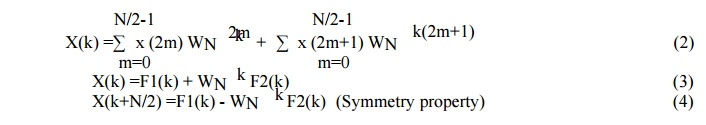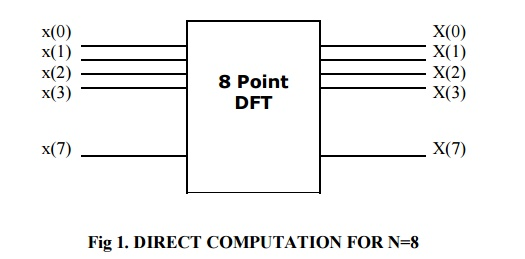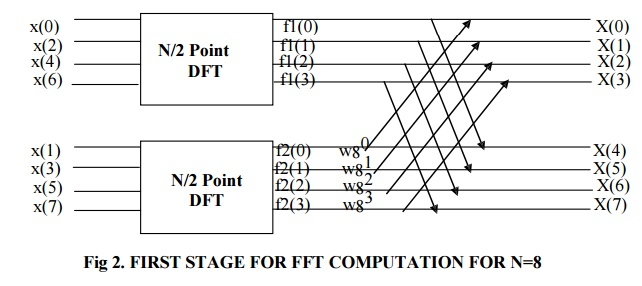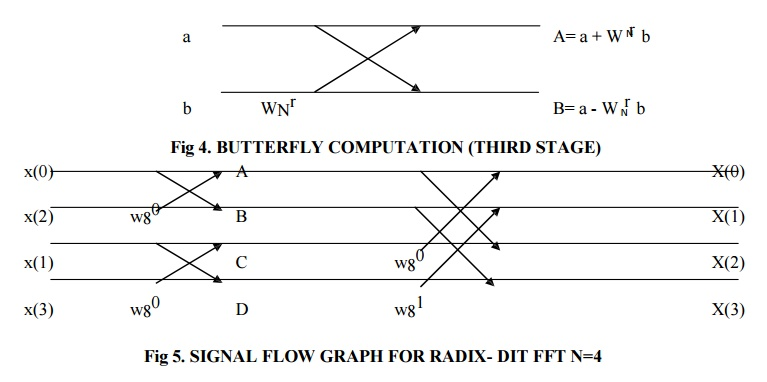## Chapter: Digital Signal Processing : Frequency Transformations

There are three properties of twiddle factor WN

DECIMATION IN TIME (DITFFT)

There are three properties of twiddle factor WNN point sequence x(n) be splitted into two N/2 point data sequences f1(n) and f2(n). f1(n) contains even numbered samples of x(n) and f2(n) contains odd numbered samples of x(n). This splitted operation is called decimation. Since it is done on time domain sequence it is called “Decimation in Time”. Thus

f1(m)=x(2m)         where n=0,1,………….N/2-1

f2(m)=x(2m+1)    where n=0,1,………….N/2-1

N point DFT is given asSince the sequence x(n) is splitted into even numbered and odd numbered samples, thusFig 1 shows that 8-point DFT can be computed directly and hence no reduction in computation.Study Material, Lecturing Notes, Assignment, Reference, Wiki description explanation, brief detail
Digital Signal Processing : Frequency Transformations : Radix-2 FFT Algorithms |